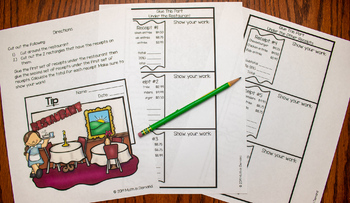### Distributive Property and Combining Like Terms Halloween Hidden Message Activity

This is a FREE resource where students will be simplifying 20 expressions by applying the distributive property and combining like terms. Students will cut out their problems and glue onto the other page according to their answers. A hidden spooky message will be revealed! This is a perfect activity individually or in small groups. The message helps this activity be self assessing!There is also 2 versions included! The second version is color coded.### Calculating Tip and Total Restaurant Bill Cut & Paste Activity

Students will be calculating the tip and total of 6 restaurant bills. There are 2 versions: (1) Involves less cutting & pasting because students will write in the tip and total on the receipts, and (2) cut & paste version where students cut out the bottom of 6 receipts and glue the total according to the receipt that they belong to.These look great on bulletin boards!### One-Step Equations Dominoes Activity with Negatives 7th Grade Math

Included is a cut and paste domino activity on one-step equations. Students will be solving 14 problems that involve solving one-step equations. This is a great activity for students to work individually, in pairs, or in a small group. You can decide if you would like students to color their dominoes or you can print the dominoes on colored paper.Equations I also have a BUNDLE that includes this activity and 20 other math dominoes activities.### Solving Equations Practice Pointers (One-Step, Two-Step, & Multi-Step Equations)

My "Solving Equations Practice Pointers" consist of 3 practice problems for solving one-step equations, solving two-step equations, and solving multi-step equations (9 problems total). The "1 pointer" is easiest, "2 pointer" is harder, and the "3 pointer" is the hardest. Students will need scissors and glue (or stapler) in order to construct the Practice Pointers book.There are 2 versions: (1) fill-in and (2) answer key. You can decide which version you would rather use.I love this book because it makes a GREAT study tool and students can use it as an example on how to solve similar math problems. You can print each concept on colored paper so that each page pops!### Operations on Integers Mystery Halloween Picture

This bundle includes 2 of my Halloween resources: (1) Adding & Subtracting Integers and (2) Multiplying & Dividing Integers. Each resource comes with a PAPER & DIGITAL copy (Google Slides).### Rational or Irrational Coloring Worksheet FREE

Students will be coloring or shading in the boxes that have rational numbers. The boxes with irrational numbers will be left white. This would make a great practice worksheet or review on rational and irrational numbers.### Slope-Intercept Form on a Graph Halloween Stained Glass Activities

Students will be graphing 9 linear equations on a graph by plotting the y-intercept and slope. After plotting all 9 lines, they will color each section according to the given color code. There is a total of 3 pages (27 linear equations). The first page includes spiders, the second page is bats, and the third page is ghosts.Don't have time to color? I have also included an alternative version that involves no coloring!This is a perfect activity for 8th grade math, 9th grade math, or any Algebra class learning about slope-intercept form.

### Mean, Median, Mode, and Range Card Sort Activity

This activity has 16 cards on mean, median, mode, and range. Students will solve 3 problems by finding the mean, median, mode, and range. After solving the problems, students will cut out the cards and place them according to the problem. This activity works well in groups of 2-4 and can be laminated so that you can use it year after year. You could also have students glue it into their interactive notebooks.CLICK HERE to check out my Math Card Sort Activity Bundle. It includes this resource and many other card sort activities in one bundle for a great price!### Least Common Multiple and Greatest Common Factor Math Interactive Notebook

This freebie is on least common multiples and and greatest common factors. It is a great addition to math interactive notebooks.If you enjoy this freebie, please check out my math interactive notebooks. I offer 6th through Algebra, and they have the same template as this freebie.FREE, FREE, FREE!!### Pythagorean Theorem Pool Table Activity

Included is 12 task cards on the Pythagorean Theorem. Students will determining the distance between two pool balls. In addition, students will need the distance between a pool ball and the pocket. This is a great activity for students working in groups of 3-4. Also, this activity can be laminated and used year after year.